# Order from Order: 3D Fractals with Cylindrical Symmetry

3D Fractals with Cylindrical Symmetry plotted sequentially

3D Fractals with cylindrical symmetry are obtained by a complete rotation of a 2D fractal endowed by a symmetry axis around this latter. Upper and lower bounds have been imposed to the escape rate of the iterated summation law in order to evidence the internal details of each fractal structure.Mandelbrot 2d (carved)

POV Ray 3.7 codeJulia 2d (carved, c = -0.7454294)

POV Ray 3.7 code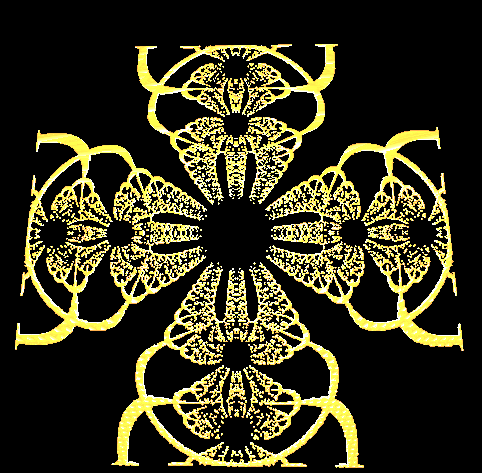Newton method 2d (carved)

POV Ray 3.7 codeMandelbrot 3D Open Section

(Cylindrical symmetry)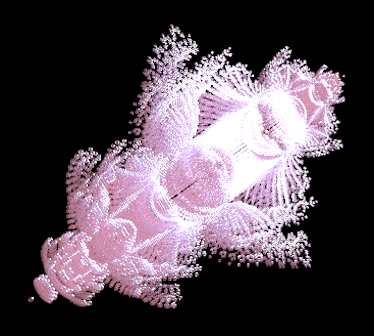Julia 3D Open Section

(Cylindrical symmetry)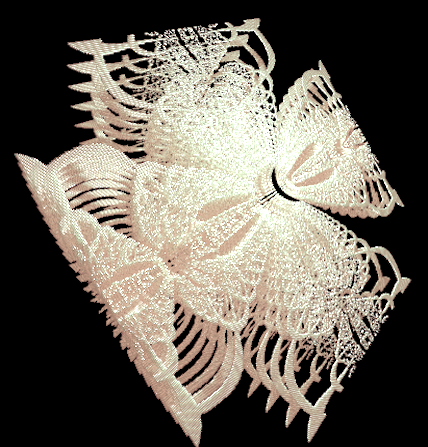Newton method 3D Open Section

(Cylindrical symmetry)Mandelbrot 3D Partial Sections

(Cylindrical symmetry)

View animationJulia 3D Partial Sections

(Cylindrical symmetry)

View animation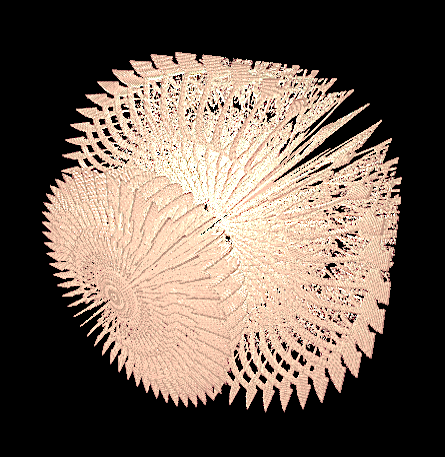Newton method 3D Partial Sections

(Cylindrical symmetry)

View animationMandelbrot 3D Closed

(Cylindrical symmetry)

POV Ray 3.7 code

View animationJulia 3D Closed

(Cylindrical symmetry)

POV Ray 3.7 code

View animation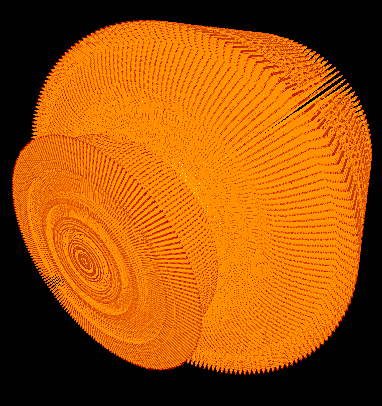Newton method 3D CloseD

(Cylindrical symmetry)

POV Ray 3.7 code

View animation